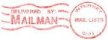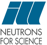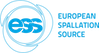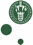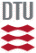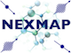McStas: Filter_gen Component

# The `Filter_gen` Component

This components may either set the flux or change it (filter-like), using an external data filename.

## Identification

• Author: E. Farhi
• Origin: ILL
• Date: Dec, 15th, 2002
• Version: \$Revision\$

## Description

```This component changes the neutron flux (weight) in order to match
a reference table in a filename.
Typically you may set the neutron flux (source-like), or multiply it
using a transmission table (filter-like).
The component may be placed after a source, in order to e.g.
simulate a real source from a reference table, or used as a filter (BeO)
or as a window (Al). The behaviour of the component is
specified using the 'options' parameter, or from the filename itself (see below)
If the thickness for the transmission data filename D was t0, and a different
thickness t1 would be required, then the resulting transmission is:
D^(t1/t0).
You may use the 'thickness' and 'scaling' parameter for that purpose.

File format:
This filename may be of any 2-columns free format (k[Angs-1],p), (omega[meV],p)
and (lambda[Angs],p) where p is the weight. The type of the filename may be
written explicitely in the filename, as a comment, or using the 'options'
parameter.
Non mumerical content in filename is treated as comment (e.g. lines starting
with '#' character).
A table rebinning and linear interpolation are performed.

EXAMPLE : in order to simulate a PG filter, using the lib/data/HOPG.trm file
Filter_gen(xwidth=.1 yheight=.1, filename="HOPG.trm")
A Sapphire filter, using the lib/data/Al2O3_sapphire.trm file
Filter_gen(xwidth=.1 yheight=.1, filename="Al2O3_sapphire.trm")
A Berylium filter, using the lib/data/Be.trm file
Filter_gen(xwidth=.1 yheight=.1, filename="Be.trm")
an other possibility to simulate a Be filter is to use the PowderN component:
PowderN(xwidth=.1, yheight=.1, zdepth=.1, reflections="Be.laz", p_inc=1e-4)

in this filename, the comment line
# wavevector multiply
sets the behaviour of the component. One may as well have used
options="wavevector multiply"
in the component instance parameters.

```

## Input parameters

Parameters in boldface are required; the others are optional.
Name Unit Description Default
filename str name of the filename to look at (first two columns data). Data D should rather be sorted (ascending order) and monotonic filename may contain options (see below) as comment 0
options str string that can contain: "[ k p ]" or "wavevector" for filename type, "[ omega p]" or "energy", "[ lambda p ]" or "wavelength", "set" to set the weight according to the table,"multiply" to multiply (instead of set) the weight by factor,"add" to add to current flux,"verbose" to display additional informations. 0
xmin m dimension of filter -0.05
xmax m dimension of filter 0.05
ymin m dimension of filter -0.05
ymax m dimension of filter 0.05
xwidth m Width/diameter of filter). Overrides xmin,xmax. 0
yheight m Height of filter. Overrides ymin,ymax. 0
thickness 1 relative thickness. D = D^(thickness). 1
scaling 1 scaling factor. D = D*scaling. 1
verbose 0

## Output parameters

Name Unit Description Default
pTable
Mode_Table
Type_Table